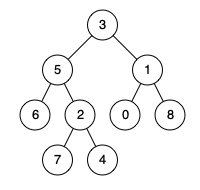# 236. Lowest Common Ancestor of a Binary Tree

Given a binary tree, find the lowest common ancestor (LCA) of two given nodes in the tree.

According to the definition of LCA on Wikipedia: “The lowest common ancestor is defined between two nodes p and q as the lowest node in T that has both p and q as descendants (where we allow a node to be a descendant of itself).”

Given the following binary tree:  root = [3,5,1,6,2,0,8,null,null,7,4]Example 1:

``````Input: root = [3,5,1,6,2,0,8,null,null,7,4], p = 5, q = 1
Output: 3
Explanation: The LCA of nodes 5 and 1 is 3.
``````

Example 2:

``````Input: root = [3,5,1,6,2,0,8,null,null,7,4], p = 5, q = 4
Output: 5
Explanation: The LCA of nodes 5 and 4 is 5, since a node can be a descendant of itself according to the LCA definition.
``````

Note:

• All of the nodes’ values will be unique.
• p and q are different and both values will exist in the binary tree.

- 若p和q分别位于左右子树中，那么对左右子结点调用递归函数，会分别返回p和q结点的位置，而当前结点正好就是p和q的最小共同父结点，直接返回当前结点即可，这就是题目中的例子1的情况。

- 若p和q同时位于左子树，这里有两种情况，一种情况是 left 会返回p和q中较高的那个位置，而 right 会返回空，所以最终返回非空的 left 即可，这就是题目中的例子2的情况。还有一种情况是会返回p和q的最小父结点，就是说当前结点的左子树中的某个结点才是p和q的最小父结点，会被返回。

- 若p和q同时位于右子树，同样这里有两种情况，一种情况是 right 会返回p和q中较高的那个位置，而 left 会返回空，所以最终返回非空的 right 即可，还有一种情况是会返回p和q的最小父结点，就是说当前结点的右子树中的某个结点才是p和q的最小父结点，会被返回，写法很简洁，代码如下：

``````class Solution {
public:
TreeNode* lowestCommonAncestor(TreeNode* root, TreeNode* p, TreeNode* q) {
if (!root || p == root || q == root) return root;
TreeNode *left = lowestCommonAncestor(root->left, p, q);
TreeNode *right = lowestCommonAncestor(root->right, p , q);
if (left && right) return root;
return left ? left : right;
}
};
``````

``````class Solution {
public:
TreeNode* lowestCommonAncestor(TreeNode* root, TreeNode* p, TreeNode* q) {
if (!root || p == root || q == root) return root;
TreeNode *left = lowestCommonAncestor(root->left, p, q);
if (left && left != p && left != q) return left;
TreeNode *right = lowestCommonAncestor(root->right, p , q);
if (left && right) return root;
return left ? left : right;
}
};
``````

Github 同步地址：

https://github.com/grandyang/leetcode/issues/236

Lowest Common Ancestor of a Binary Search Tree

https://leetcode.com/problems/lowest-common-ancestor-of-a-binary-tree/

https://leetcode.com/problems/lowest-common-ancestor-of-a-binary-tree/discuss/65225/4-lines-C++JavaPythonRuby

LeetCode All in One 题目讲解汇总(持续更新中…)

 微信打赏Venmo 打赏×

Help us with donation